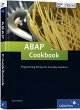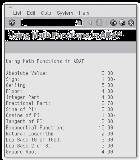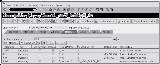# Advanced ABAP operations with elementary data types

## In this book chapter excerpt, discover some ABAP advanced operations that can be performed using elementary data. You'll also learn how to use these features in your ABAP programs.In this excerpt from the ABAP Cookbook, a publication of SAP Press, you'll discover some SAP ABAP advanced operations that can be performed using elementary data. You'll also learn how to use these features in your ABAP programs.

Working with Numbers, Dates, and Bytes

One of the nice things about working with an advanced programming language like ABAP is that you don’t often have to worry about how that data is represented behind the scenes at the bits and bytes level; the language does such a good job of abstracting data that it becomes irrelevant. However, if you do come across a requirement that compels you to dig a little deeper, you’ll find that ABAP also has excellent support for performing more advanced operations with elementary data types. In this chapter, we investigate some of these operations and show you techniques for using these features in your programs.

2.1 Numeric Operations

Whether it’s keeping up with a loop index or calculating entries in a balance sheet, almost every ABAP program works with numbers on some level. Typically, whenever we perform operations on these numbers, we use basic arithmetic operators such as the + (addition), - (subtraction), * (multiplication), or / (division) operators. Occasionally, we might use the MOD operator to calculate the remainder of an integer division operation, or the ** operator to calculate the value of a number raised to the power of another. However, sometimes we need to perform more advanced calculations. If you’re a mathematics guru, then perhaps you could come up with an algorithm to perform these advanced calculations using the basic arithmetic operators available in ABAP. For the rest of us mere mortals, ABAP provides an extensive set of mathematics tools that can be used to simplify these requirements. In the next two sections, we’ll examine these tools and see how to use them in your programs.

2.1.1 ABAP Math Functions

ABAP provides many built-in math functions that you can use to develop advanced mathematical formulas as listed in Table 2.1. In many cases, these functions can be called using any of the built-in numeric data types in ABAP (e.g., the I, F, and P data types). However, some of these functions require the precision of the floating point data type (see Table 2.1 for more details). Because ABAP supports implicit type conversion between numeric types, you can easily cast non-floating point types into floating point types for use within these functions.

 Function Supported Numeric Types Description abs (All) Calculates the absolute value of the provided argument. sign (All) Determines the sign of the provided argument. If the sign is positive, the function returns 1; if it’s negative, it returns -1; otherwise, it returns 0. ceil (All) Calculates the smallest integer value that isn’t smaller than the argument.
 floor (All) Calculates the largest integer value that isn’t larger than the argument. trunc (All) Returns the integer part of the argument. frac (All) Returns the fractional part of the argument. cos, sin, tan F Implements the basic trigonometric functions.
 acos, asin, atan F Implements the inverse trigonometric functions. cosh, sinh, tanh F Implements the hyperbolic trigonometric functions. exp F Implements the exponential function with a base e ≈ 2.7182818285. log F Implements the natural logarithm function.
 log10 F Calculates a logarithm using base 10. sqrt F Calculates the square root of a number.

Table 2.1 ABAP Math Functions

The report program ZMATHDEMO shown in Listing 2.1 contains examples of how to call the math functions listed in Table 2.1 in an ABAP program. The output of this program is displayed in Figure 2.1.

REPORT zmathdemo.

START-OF-SELECTION. CONSTANTS: CO_PI TYPE f VALUE '3.14159265'. DATA: lv_result TYPE p DECIMALS 2.

lv_result = abs( -3 ). WRITE: / 'Absolute Value: ', lv_result.

lv_result = sign( -12 ). WRITE: / 'Sign: ', lv_result.

lv_result = ceil( '4.7' ). WRITE: / 'Ceiling: ', lv_result.

lv_result = floor( '4.7' ). WRITE: / 'Floor: ', lv_result.

lv_result = trunc( '4.7' ). WRITE: / 'Integer Part: ', lv_result.

lv_result = frac( '4.7' ). WRITE: / 'Fractional Part: ', lv_result.

lv_result = sin( CO_PI ). WRITE: / 'Sine of PI: ', lv_result.

lv_result = cos( CO_PI ). WRITE: / 'Cosine of PI: ', lv_result.

lv_result = tan( CO_PI ). WRITE: / 'Tangent of PI: ', lv_result.

lv_result = exp( '2.3026' ). WRITE: / 'Exponential Function:', lv_result.

lv_result = log( lv_result ). WRITE: / 'Natural Logarithm: ', lv_result.

lv_result = log10( '1000.0' ). WRITE: / 'Log Base 10 of 1000: ', lv_result.

\lv_result = log( 8 ) / log( 2 ). WRITE: / 'Log Base 2 of 8: ', lv_result.

lv_result = sqrt( '16.0' ). WRITE: / 'Square Root: ', lv_result.

Listing 2.1 Working with ABAP Math FunctionsFigure 2.1 Output Generated by Report ZMATHDEMO

The values of the function calls can be used as operands in more complex expressions. For example, in Listing 2.1, notice how we’re calculating the value of log( 8 ). Here, we use the change of base formula log( x ) / log( b ) (where b refers to the target base, and x refers to the value applied to the logarithm function) to derive the base 2 value. Collectively, these functions can be combined with typical math operators to devise some very complex mathematical formulas.

2.1.2 Generating Random Numbers

Computers live in a logical world where everything is supposed to make sense. Whereas this characteristic makes computers very good at automating many kinds of tasks, it can also make it somewhat difficult to model certain real-world phenomena. Often, we need to simulate imperfection in some form or another. One common method for achieving this is to produce randomized data using random number generators. Random numbers are commonly used in statistics, cryptography, and many kinds of scientific applications. They are also used in algorithm design to implement fairness and to simulate useful metaphors applied to the study of artificial intelligence (e.g., genetic algorithms with randomized mutations, etc.).

SAP provides random number generators for all of the built-in numeric data types via a series of ABAP Objects classes. These classes begin with the prefix CL_ABAP_ RANDOM (e.g., CL_ABAP_RANDOM_FLOAT, CL_ABAP_RANDOM_INT, etc.). Though none of these classes inherit from the CL_ABAP_RANDOM base class, they do use its features behind the scenes using a common OO technique called composition. Composition basically implies that one class delegates certain functionality to an instance of another class. The UML class diagram shown in Figure 2.2 shows the basic structure of the provided random number generator classes.

 CL_ABAP_RANDOM_* + CREATE ( ) + GET_NEXT( )

Figure 2.2 Basic UML Class Diagram for Random Number Generators

Unlike most classes where you create an object using the CREATE OBJECT statement, instances of random number generators must be created via a call to a factory class method called CREATE(). The signature of the CREATE() method is shown in Figure 2.3. Here, you can see that the method defines an importing parameter called SEED that seeds the pseudo-random number generator algorithm that is used behind the scenes to generate the random numbers. In a pseudo-random number generator, random numbers are generated in sequence based on some calculation performed using the seed. Thus, a given seed value causes the random number generator to generate the same sequence of random numbers each time.

The CREATE() method for class CL_ABAP_RANDOM_INT also provides MIN and MAX parameters that can place limits around the random numbers that are generated (e.g., a range of 1-100, etc.). The returning PRNG parameter represents the generated random number generator instance. Once created, you can begin retrieving random numbers via a call to the GET_NEXT() instance method.Figure 2.3 Signature of Class Method CREATE()

To demonstrate how these random number generator classes work, let’s consider an example program. Listing 2.2 contains a simple report program named ZSCRAMBLER that defi nes a local class called LCL_SCRAMBLER. The LCL_SCRAMBLER class includes an instance method SCRAMBLE() that can be used to randomly scramble around the characters in a string. This primitive implementation creates a random number generator to produce random numbers in the range of [0... {String Length}]. Perhaps the most complex part of the implementation is related to the fact that random number generators produce some duplicates along the way. Therefore, we have to make sure that we haven’t used the randomly generated number previously to make sure that each character in the original string is copied into the new one.

REPORT zscrambler.

CLASS lcl_scrambler DEFINITION. PUBLIC SECTION. METHODS: scramble IMPORTING im_value TYPE clike RETURNING VALUE(re_svalue) TYPE string EXCEPTIONS cx_abap_random.

PRIVATE SECTION. CONSTANTS: CO_SEED TYPE i VALUE 100.

TYPES: BEGIN OF ty_index, index TYPE i, END OF ty_index. ENDCLASS.

CLASS lcl_scrambler IMPLEMENTATION. METHOD scramble.*

Method-Local Data Declarations: DATA: lv_length TYPE i, lv_min TYPE i VALUE 0, lv_max TYPE i, lo_prng TYPE REF TO cl_abap_random_int, lv_index TYPE i, lt_indexes TYPE STANDARD TABLE OF ty_index. FIELD-SYMBOLS: <lfs_index> LIKE LINE OF lt_indexes.

* Determine the length of the string as this sets the * bounds on the scramble routine: lv_length = strlen( im_value ). lv_max = lv_length - 1.

* Create a random number generator to return random * numbers in the range of 1..{String Length}: CALL METHOD cl_abap_random_int=>create EXPORTING seed = CO_SEED min = lv_min max = lv_max RECEIVING prng = lo_prng.

* Add the characters from the string in random order to * the result string: WHILE strlen( re_svalue ) LT lv_length. lv_index = lo_prng->get_next( ). READ TABLE lt_indexes TRANSPORTING NO FIELDS WITH KEY index = lv_index. IF sy-subrc EQ 0. CONTINUE. ENDIF.

CONCATENATE re_svalue im_value+lv_index(1) INTO re_svalue. APPEND INITIAL LINE TO lt_indexes ASSIGNING <lfs_index>. <lfs_index>-index = lv_index. ENDWHILE. ENDMETHOD. ENDCLASS.

START-OF-SELECTION. * Local Data Declarations: DATA: lo_scrambler TYPE REF TO lcl_scrambler, lv_scrambled TYPE string.

* Use the scrambler to scramble around a word: CREATE OBJECT lo_scrambler. lv_scrambled = lo_scrambler->scramble( 'Andersen' ). WRITE: / lv_scrambled.

Listing 2.2 Using Random Number Generators in ABAP

Obviously, a simple scrambler routine like the one shown in Listing 2.2 isn’t production quality. Nevertheless, it does give you a glimpse of how you can use random number generators to implement some interesting algorithms. As a reader exercise, you might think about how you could use random number generators to implement an UNSCRAMBLE() method to unscramble strings generated from calls to method SCRAMBLE().